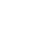Back to the list

# How to use a SUMIF in Microsoft Excel

## SUMIF

Is a function in Microsoft Excel that allows you to sum a range of cells based on a specific condition. It can be used to add up all values that meet a certain criteria, such as finding the total sales for a particular region. The syntax for SUMIF is as follows: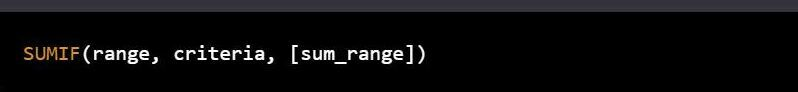##### SUMIF(range, criteria, [sum_range])

• range is the range of cells to be evaluated for the criteria.
• criteria is the condition that must be met for a cell to be included in the sum. It can be a value, a cell reference, or a text string.
• sum_range is an optional argument that specifies the range of cells to be summed. If not specified, the range argument is used for both the evaluation and summing.

Here's an example of how to use SUMIF in Microsoft Excel:
Imagine you have a worksheet with a list of sales by region, as follows: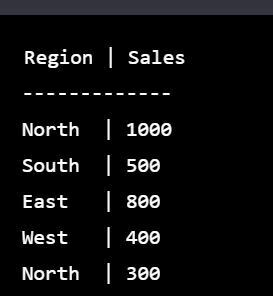To find the total sales for the North region, you can use the following formula: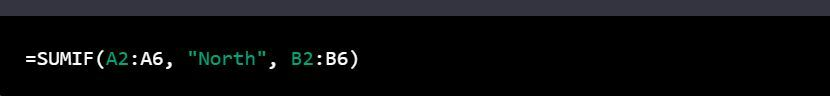This formula will evaluate the range A2:A6 for cells that meet the criteria "North", and then sum the corresponding values in the sum_range B2:B6. The result will be 1300.

Another example is to sum the sales for values greater than 500. The formula would be: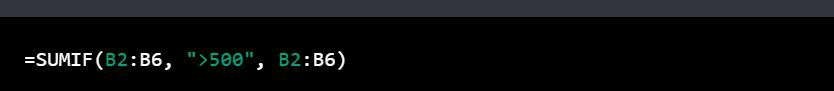In this example, the criteria is a logical expression (">500"). The function will evaluate the range B2:B6 for values greater than 500 and then sum the corresponding values in the sum_range B2:B6. The result will be 2600.